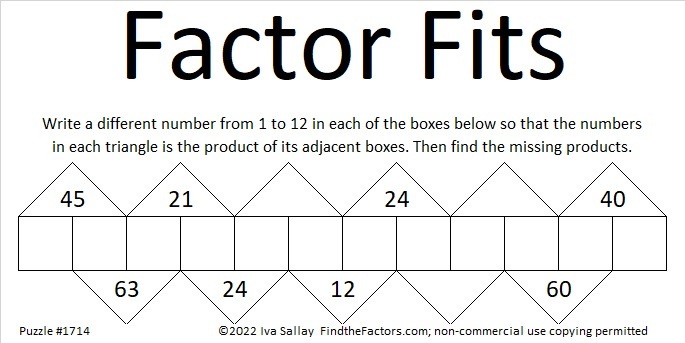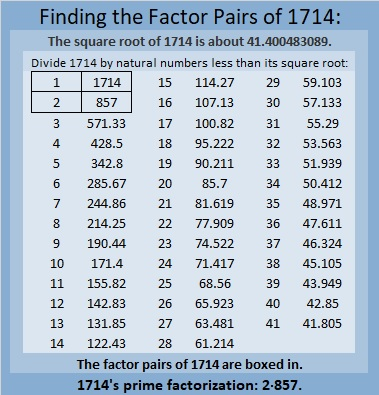# 1714 Will the Factors in This Puzzle Give You Fits?

Contents

### Today’s Puzzle:

12 and 24 have several common factors, but only one of them works in this puzzle. Will it be 2, 3, 4, 6, or 12?

What about 40 and 60’s common factors?

Don’t guess which factor to use. Start elsewhere in the puzzle where there’s only one possible common factor. Then use logic to eliminate some of the factor possibilities for 12, 24 and 40, 60. You will have to think, but it won’t be too difficult.### Factors of 1714:

• 1714 is a composite number.
• Prime factorization: 1714 = 2 × 857.
• 1714 has no exponents greater than 1 in its prime factorization, so √1714 cannot be simplified.
• The exponents in the prime factorization are 1 and 1. Adding one to each exponent and multiplying we get (1 + 1)(1 + 1) = 2 × 2 = 4. Therefore 1714 has exactly 4 factors.
• The factors of 1714 are outlined with their factor pair partners in the graphic below.### More About the Number 1714:

1714 is the sum of two squares:
33² + 25² = 1714.

1714 is the hypotenuse of a Pythagorean triple:
464-1650-1714, calculated from 33² – 25², 2(33)(25), 33² + 25².
It is also 2 times (232-825-857).

This site uses Akismet to reduce spam. Learn how your comment data is processed.